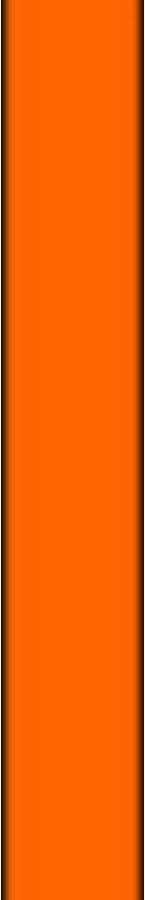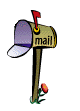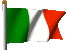The Binary System Only two "symbols" are necessary to represent an information (a numbre, a letter, etc. ) For example the Morse Alphabet, created in the second half of 1800 for the informations' telegraphic transmission, is based on two symbols "point" and "line", which opportunely combined, represent all the letters and all the numbers. This approach is exploited in the calculation machine as well, which is a machine based on some elementary members that can have two different conditions only : in a circuit the electricity passes or doesn't pass, an electronic tube is on or off, a ferromagnetic ring is magnetized on a side or on the opposit one. To allow the calculation machine to rekord the informations, the two possible conditions of its components are shown conventionally by 1 and 0, and the numbers, the letters and the special characters are represented by the combination of a set of 1 and 0. This code is called "binary" and the two symbols 1 and 0 are also called "Bit" (Binary Digit). The numerical system normally used by man is the "decimal" one, which adopts 10 symbols. In a figure, the position occupied by the digits represents the unity, the tens , the hundreds,etc., it means the "growing power of ten". The numeric binary system, which is adopted by the calculation machines, uses only two symbols (1 and 0) and the position which is occupied by them, represents the "growing power of two". When it moves on the left of a column, each digit's value doesn't increase of ten times, as in the decimal system, but of two times. In both the decimal system and the binary one, in one number the value of each digit depends on the position taken in the number's ambit according to the formula :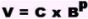The value (V) is given by the digit (C) multiplied the base (B) raised to the position (P) which is occupied by the digit in the number's ambit, beginning from the 0 position and running the number from right towards left. For example the binary 1001 is the decimal 9. Besides the numbers, even the letters, the punctuation marks and some other symbols with a special meaning can be represented by the two digits 1 and 0, through the use of codes which are based on the basic binary system. As 1 and 0 means for the machine the presence or absence of an electric impulse, it is possible communicate to the calculation machine through electric marks, all the informations and the necessary instructions to execute the requested operations. The adoption of the numerical binary system has more advantages for the calculation machine than the decimal system, infact it is possible to distnguish an electronic mark only between the possible two, rather than among ten, and it is also possible to use the rules of the binary arithmetic which is more simple than the decimal one. To execute the various arithmetical and logic operations the calculation machine bases itself on few and simple rules known as "Boole's Algebra", from the name of the English mathematician who formulated them for the binary systems in the second half of 1800. The machine's calculus circuits connect various electric impulses which are received to respect the binary rules of the sum, of the multiplication etc. The multiplications are executed by the calculation machine like a series of following , sums, the subtractions and the divisions are trasformed into sums by special "rules": using the binary system, the machine doesn't execute anything more complicated than 1 + 1. Executing the subtractions, the calculation machine is able to develop the logic function of the decision, which is nothing else than another comparison between two sizes, it means a subtraction. According to this subtraction's results, which can be also a positive number, a negative number or nought, the calculation machine can be predisposed to execute alternate operations.
```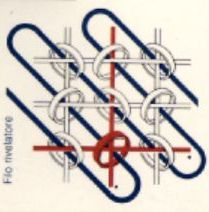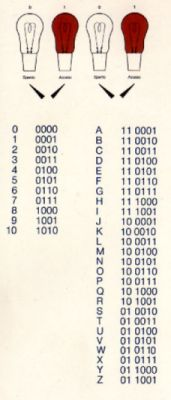```

Ferromagnetic Rings
Binary Code